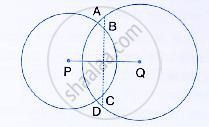Share

In the Following Figure, the Line Abcd is Perpendicular to Pq; Where P and Q Are the Centres of the Circles. Show That: (I) Ab = Cd, (Ii) Ac = Bd. - ICSE Class 10 - Mathematics

ConceptChord Properties - the Perpendicular to a Chord from the Center Bisects the Chord (Without Proof)

Question

In the following figure, the line ABCD is perpendicular to PQ; where P and Q are the centres of
the circles. Show that:
(i) AB = CD,
(ii) AC = BD.Solution

In the circle with centre Q, QO ⊥ AD
∴ OA= OD        …………… (1)
(Perpendicular drawn from the centre of a circle to a chord bisects it)
In the circle with centre P, PO ⊥ BC
∴ OB=OC          ……………..(2)
(Perpendicular drawn from the centre of a circle to a chord bisects it)
(i)
(1) – (2) Gives,
AB = CD ……………(3)

(ii) Adding BC to both sides of equation (3)
AB+BC = CD+BC
⇒ AC = BD

Is there an error in this question or solution?
Solution In the Following Figure, the Line Abcd is Perpendicular to Pq; Where P and Q Are the Centres of the Circles. Show That: (I) Ab = Cd, (Ii) Ac = Bd. Concept: Chord Properties - the Perpendicular to a Chord from the Center Bisects the Chord (Without Proof).
S